Surrogate Based Optimization Design of Six-Element Yagi-Uda Antenna

This example optimizes a 6-element Yagi-Uda antenna for both directivity and 50 $\Omega$ input match using a global optimization technique called surrogate optimization. The radiation patterns and input impedance of antennas are sensitive to the parameters that define their shapes. The multidimensional surface over which such optimizations must be performed have multiple local optima. This makes the task of finding the right set of parameters satisfying the optimization goals particularly challenging and requires the use of global optimization techniques. This Yagi-Uda antenna is intended for operation as part of a repeater station in a HAM radio setup.

Design Parameters

Choose initial design parameters in the center of the VHF band.

fc = 144.5e6;
wirediameter = 12e-3;
c = physconst('lightspeed');
lambda = c/fc;
Z0 = 50;
BW = 0.015*fc;
fmin = fc - 2*(BW);
fmax = fc + 2*(BW);
Nf = 101;
freq = linspace(fmin,fmax,Nf);

Create Yagi-Uda Antenna

The driven element for this Yagi-Uda antenna is a dipole. This is a standard exciter for such an antenna. Adjust the length and width parameters of the dipole. Since we model cylindrical structures as equivalent metal strips, the width is calculated using a utility function available in the Antenna Toolbox™. The length is chosen to be approximately $\lambda /2$ at the design frequency.

d = dipole;
d.Length = 0.982.*(lambda/2);
d.Width = cylinder2strip(wirediameter/2);
d.Tilt = 90;
d.TiltAxis = 'Y';

Create a Yagi-Uda antenna with the exciter as the dipole. Set the number of directors to four. The choices for the lengths of the elements and spacing between the elements are an initial guess and will serve as a start point for the optimization procedure. Show the initial design.

Numdirs = 4;
refLength = 0.25;
dirLength = [0.940 0.910 0.850 0.830];
refSpacing = 0.35;
dirSpacing = [0.15 0.2 0.3 0.3 ];
initialdesign = [refLength refSpacing dirSpacing].*lambda;
yagidesign = yagiUda;
yagidesign.Exciter = d;
yagidesign.NumDirectors = Numdirs;
yagidesign.ReflectorLength = refLength;
yagidesign.DirectorLength = dirLength;
yagidesign.ReflectorSpacing = refSpacing*lambda;
yagidesign.DirectorSpacing = dirSpacing*lambda;
show(yagidesign)Plot Radiation Pattern at Design Frequency

Prior to executing the optimization process, plot the radiation pattern for the initial guess in 3D.

pattern(yagidesign,fc);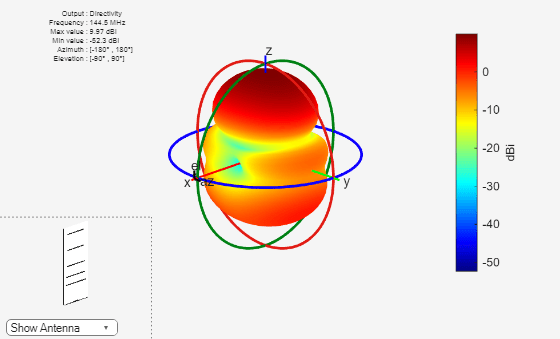This initial Yagi-Uda antenna does not have a higher directivity in the preferred direction, meaning at zenith (elevation = 90 deg) and is therefore a poorly designed radiator.

Set Up Optimization

Use the following variables as control variables for the optimization:

• Reflector length (1 variable)

• Reflector spacing (1 variable)

• Director spacings (4 variables)

In terms of a single vector parameter controlVals, set

• Reflector length = controlVals(1)

• Reflector spacing = controlVals(2)

• Director spacings = controlVals(3:6)

In terms of controlVals, set an objective function that aims to have a large directivity value in the 90 degree direction, a small value in the -90 degree direction, and a large value of maximum power between the elevation beamwidth angle bounds. In addition to the directivity goal an impedance match condition is also included as a constraint. Any constraint violations will penalize the objective.

type yagi_objective_function_surrogate.m
function objectivevalue = yagi_objective_function_surrogate(y,controlVals,fc,BW,ang,Z0,constraints)
% YAGI_OBJECTIVE_FUNCTION returns the objective for a 6 element Yagi
% OBJECTIVE_VALUE =
% YAGI_OBJECTIVE_FUNCTION(Y,CONTROLVALS,FREQ,ANG,Z0,constraints), assigns
% the appropriate parasitic dimensions, CONTROLVALS to the Yagi antenna Y,
% and uses the frequency FREQ, angle pair,ANG, reference impedance Z0 and
% the constraints to calculate the objective function value.

% The YAGI_OBJECTIVE_FUNCTION function is used for an internal example.
% Its behavior may change in subsequent releases, so it should not be
% relied upon for programming purposes.

% Copyright 2018 The MathWorks, Inc.

y.ReflectorLength = controlVals(1);
y.ReflectorSpacing = controlVals(2);
y.DirectorSpacing = controlVals(3:end);

% Unpack constraints
Gmin = constraints.Gmin;
Gdev = constraints.Gdeviation;
FBmin = constraints.FBmin;
S11min = constraints.S11min;
K = constraints.Penalty;

% Calculate antenna port and field parameters
output = analyzeAntenna(y,fc,BW,ang,Z0);

% Form objective function
output1 = output.MaxDirectivity+output.MismatchLoss;    % Directivity/Gain at zenith
Gain1 = output.MaxDirectivity1+output.MismatchLoss1;    % Directivity/Gain at zenith
Gain2 = output.MaxDirectivity2+output.MismatchLoss2;    % Directivity/Gain at zenith
% Gain constraint, e.g. G > 10
c1 = 0;
if output1<Gmin
c1 = abs(Gmin-output1)+abs(Gain1-Gmin)+abs(Gain2-Gmin);
end

% Gain deviation constraint, abs(G-Gmin)<0.1;
c1_dev = 0;
c1_dev_temp = 0;
c1_dev_temp1 = 0;
c1_dev_temp2 = 0;
if abs(output1-Gmin)>Gdev
c1_dev_temp = -Gdev + abs(output1-Gmin);
end
if abs(Gain1-Gmin)>Gdev
c1_dev_temp1 = -Gdev + abs(Gain1-Gmin);
end
if abs(Gain2-Gmin)>Gdev
c1_dev_temp2 = -Gdev + abs(Gain2-Gmin);
end
c1_dev = (c1_dev_temp+c1_dev_temp1+c1_dev_temp2)/3;

% Front to Back Ratio constraint, e.g. F/B > 15
c2 = 0;
% if output.FB < FBmin
%     c2 = FBmin-output.FB;
% end
c2 = (abs(FBmin-output.FB)+abs(FBmin-output.FB1)+abs(FBmin-output.FB2))/3;

% Reflection Coefficient, S11 < -10
c3 = 0;
if output.S11 > S11min
c3 = -S11min + output.S11;
end

% Form the objective + constraints
objectivevalue = -output1 + max(0,(c1+c1_dev+c2+c3))*K;
end

function output = analyzeAntenna(ant,fc,BW,ang,Z0)
%ANALYZEANTENNA calculate the objective function
% OUTPUT = ANALYZEANTENNA(Y,FREQ,BW,ANG,Z0) performs analysis on the
% antenna ANT at the frequency, FC, and calculates the directivity at the
% angles specified by ANG and the front-to-back ratio. The reflection
% coefficient relative to reference impedance Z0, and impedance are
% computed over the bandwidth BW around FC.

fmin = fc - (BW/2);
fmax = fc + (BW/2);
Nf = 5;
freq = unique([fc,linspace(fmin,fmax,Nf)]);
fcIdx = freq==fc;
fcIdx1 = freq==fmin;
fcIdx2 = freq==fmax;
s = sparameters(ant,freq,Z0);
Z = impedance(ant,fc);
Z1 = impedance(ant,fmin);
Z2 = impedance(ant,fmax);
az = ang(1,:);
el = ang(2,:);
Dmax = pattern(ant,fc,az(1),el(1));
Dmax1 = pattern(ant,fmin,az(1),el(1));
Dmax2 = pattern(ant,fmax,az(1),el(1));
Dback = pattern(ant,fc,az(2),el(2));
Dback1 = pattern(ant,fmin,az(2),el(2));
Dback2 = pattern(ant,fmax,az(2),el(2));
% Calculate F/B
F_by_B = Dmax-Dback;
F_by_B1 = (Dmax1-Dback1);
F_by_B2 = (Dmax2-Dback2);
% Compute S11 and mismatch loss
s11 = rfparam(s,1,1);
S11 = max(20*log10(abs(s11)));
T = mean(10*log10(1 - (abs(s11)).^2));
T1 = max(10*log10(1 - (abs(s11(fcIdx1))).^2));
T2 = max(10*log10(1 - (abs(s11(fcIdx2))).^2));
% Form the output structure
output.MaxDirectivity= Dmax;
output.BackLobeLevel = Dback;
output.MaxDirectivity1= Dmax1;
output.BackLobeLevel1 = Dback1;
output.MaxDirectivity2= Dmax2;
output.BackLobeLevel2 = Dback2;
output.FB = F_by_B;
output.FB1 = F_by_B1;
output.FB2 = F_by_B2;
output.S11 = S11;
output.MismatchLoss = T;
output.MismatchLoss1 = T1;
output.MismatchLoss2 = T2;
output.Z = Z;
output.Z1 = Z1;
output.Z2 = Z2;
end

Set bounds on the control variables.

refLengthBounds = [0.1;                         % lower bound on reflector length
0.6];                        % upper bound on reflector spacing
dirLengthBounds = [0.3 0.3 0.3 0.3;             % lower bound on director length
0.7 0.7 0.7 0.7];            % upper bound on director length
refSpacingBounds = [0.25;                       % lower bound on reflector spacing
0.65];                      % upper bound on reflector spacing
dirSpacingBounds = [0.01 0.1 0.1 0.1;           % lower bound on director spacing 0.2 0.25 0.3 0.3
0.2 0.25 0.35 0.35];        % upper bound on director spacing
exciterLengthBounds = [0.45;                    % lower bound on exciter length
0.6];                    % upper bound on exciter length
exciterSpacingBounds = [.004;
.008];
LB = [refLengthBounds(1) refSpacingBounds(1) dirSpacingBounds(1,:) ].*lambda;
UB = [refLengthBounds(2) refSpacingBounds(2) dirSpacingBounds(2,:) ].*lambda;
parameterBounds.LB = LB;
parameterBounds.UB = UB;
ang = [0 0;90 -90];                             % azimuth,elevation angles for main lobe and back lobe [az;el]

Surrogate Based Optimization

The Global Optimization Toolbox™ provides a surrogate based optimization function called surrogate. We use this function with options specified with the optimoptions function. At every iteration, plot the best value of the objective function and limit the total number of iterations to 300. Pass the objective function to the surrogate function by using an anonymous function together with the bounds and the options structure.The objective function used during the optimization process by surrogate is available in the file yagi_objective_function.

% Optimizer options
optimizer = 'Surrogate';

if strcmpi(optimizer,'PatternSearch')
optimizerparams = optimoptions(@patternsearch);
optimizerparams.UseCompletePoll = true;
optimizerparams.PlotFcns = @psplotbestf;
optimizerparams.UseParallel = true;
optimizerparams.Cache = 'on';
optimizerparams.MaxFunctionEvaluations = 1200;
optimizerparams.FunctionTolerance = 1e-2;
elseif strcmpi(optimizer,'Surrogate')
optimizerparams = optimoptions(@surrogateopt);
optimizerparams.UseParallel = true;
optimizerparams.MaxFunctionEvaluations = 600;
optimizerparams.MinSurrogatePoints = 12;
optimizerparams.InitialPoints = initialdesign;
else
error('Optimizer not supported');
end

% Antenna design parameters
designparams.Antenna = yagidesign;
designparams.Bounds = parameterBounds;

% Analysis parameters
analysisparams.CenterFrequency = fc;
analysisparams.Bandwidth = BW;
analysisparams.ReferenceImpedance = Z0;
analysisparams.MainLobeDirection = ang(:,1);
analysisparams.BackLobeDirection = ang(:,2);

% Set constraints
constraints.S11min = -15;
constraints.Gmin = 10;
constraints.Gdeviation = 0.1;
constraints.FBmin = 20;
constraints.Penalty = 75;
poolobj = gcp;
Starting parallel pool (parpool) using the 'local' profile ...
Connected to the parallel pool (number of workers: 6).
optimdesign = optimizeAntennaSurrogate(designparams,analysisparams,constraints,optimizerparams);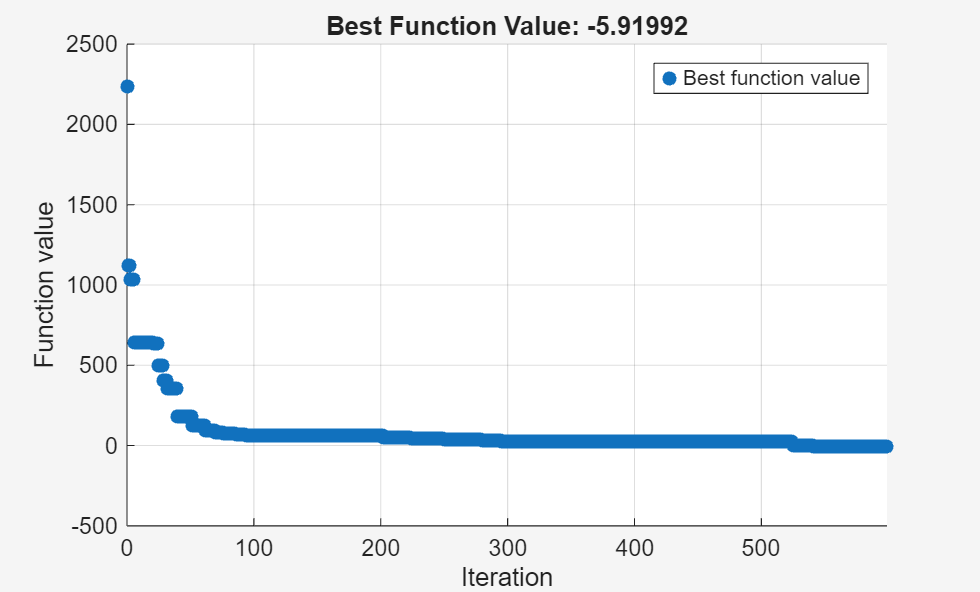surrogateopt stopped because it exceeded the function evaluation limit set by
'options.MaxFunctionEvaluations'.

Plot Optimized Pattern

Plot the optimized antenna pattern at the design frequency.

yagidesign.ReflectorLength = optimdesign(1);
yagidesign.ReflectorSpacing = optimdesign(2);
yagidesign.DirectorSpacing = optimdesign(3:6);
pattern(yagidesign,fc)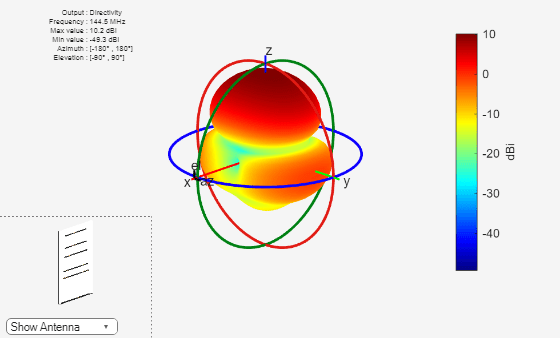E and H-Plane Cuts of Pattern

To obtain a better insight into the behavior in the two orthogonal planes, plot the normalized magnitude of the electric field in the E and H-planes, i.e. azimuth = 0 and 90 deg respectively. Enable the antenna metrics on the polar pattern plots to establish the directivity at zenith, Front-to-Back ratio, and the beamwidth in E and H-planes.

fU = fc+ BW/2;
fL = fc-BW/2;
figure;
patternElevation(yagidesign,fc,0,'Elevation',0:1:359);
pE = polarpattern('gco');
DE_fL = patternElevation(yagidesign,fL,0,'Elevation',0:1:359);
DE_fU = patternElevation(yagidesign,fU,0,'Elevation',0:1:359);
pE.MagnitudeLim = [-20 15];
pE.TitleTop = 'E-plane Directivity (dBi)';
pE.LegendLabels = {[num2str(fc./1e6), ' MHz'],[num2str(fL./1e6), ' MHz'],[num2str(fU./1e6), ' MHz']};figure;
patternElevation(yagidesign,fc,90,'Elevation',0:1:359);
pH = polarpattern('gco');
DH_fL = patternElevation(yagidesign,fL,90,'Elevation',0:1:359);
DH_fU = pattern(yagidesign,fU,90,'Elevation',0:1:359);
pH.MagnitudeLim = [-20 15];
pH.TitleTop = 'H-plane Directivity (dBi)';
pH.LegendLabels = {[num2str(fc./1e6), ' MHz'],[num2str(fL./1e6), ' MHz'],[num2str(fU./1e6), ' MHz']};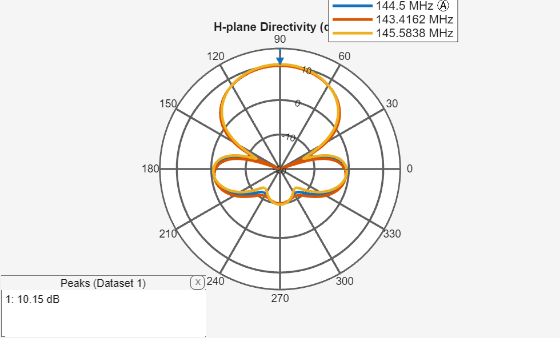The optimized design shows a significant improvement in the radiation pattern. There is higher directivity achieved in the desired direction toward zenith. The back lobe is small, resulting in a good front to back ratio for this antenna.

Input Reflection Coefficient of Optimized Antenna

The input reflection coefficient for the optimized Yagi-Uda antenna is computed and plotted relative to the reference impedance of $50\Omega$. A value of -10 dB or lower is considered as a good impedance match.

s = sparameters(yagidesign,freq,Z0);
figure;
rfplot(s);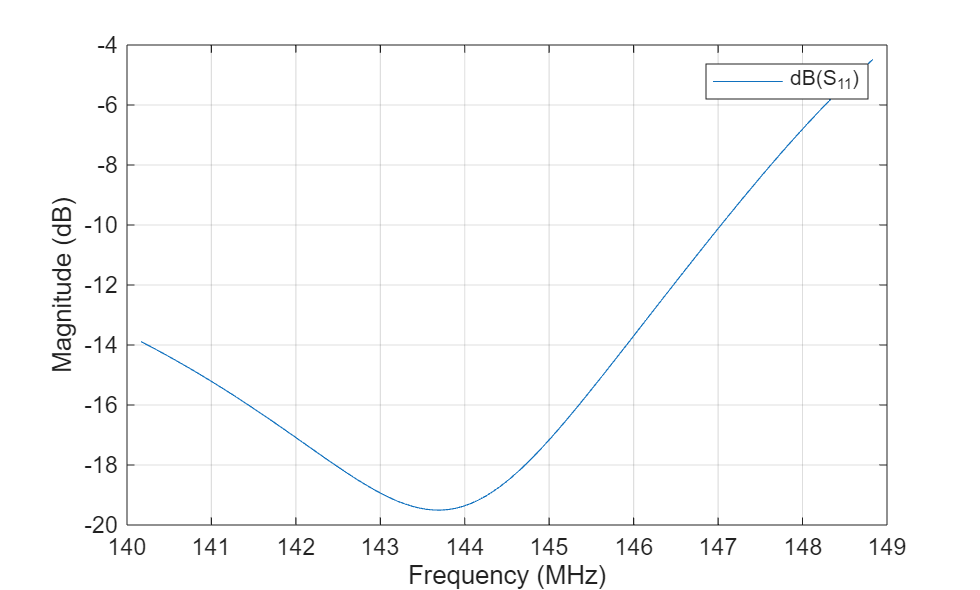Tabulating Initial and Optimized Design

Tabulate the initial design guesses and the final optimized design values.

yagiparam=  {'Reflector Length';'Reflector Spacing';'Director Spacing - 1';
'Director Spacing - 2';'Director Spacing - 3';
'Director Spacing - 4'};
initialdesign = initialdesign';
optimdesign = optimdesign';
Tgeometry = table(initialdesign,optimdesign,'RowNames',yagiparam)
Tgeometry=6×2 table
initialdesign    optimdesign
_____________    ___________

Reflector Length           0.51867          1.1327
Reflector Spacing          0.72614         0.87689
Director Spacing - 1        0.3112         0.20547
Director Spacing - 2       0.41494          0.4871
Director Spacing - 3       0.62241         0.66081
Director Spacing - 4       0.62241         0.72399

Fabricated Antennas

The optimized yagi design was fabricated. The real yagi needs a supporting element along the longitudinal axis to impart mechanical rigidity. This supporting element is called a boom and is usually manufactured out of a non-metallic material. In this case pVC tubing was used to make the boom. Note that the effect of this boom has not been modeled in the Antenna Toolbox yagiUda element. Another technique of analyzing the input match is to calculate and plot the VSWR (Voltage Standing Wave Ratio). Calculate and plot the predicted VSWR from the optimized design. The fabricated antennas VSWR was measured as well using an SWR meter. This data was saved in CSV file. Overlay the measured results with the analysis.

figure
vswr(yagidesign,freq,Z0)
hold on
plot(vswr_measured(:,1),vswr_measured(:,2),'k-.')
legend('Analysis','Measurement')
title('VSWR comparison - No coaxial cable in analysis')
ylabel('Magnitude')Model the Effect of Coaxial Cable

The coaxial cable connected to the fabricated yagi antenna is a RG-58/U with a characteristic impedance of 50 $\Omega$. Create a model of this coaxial cable using RF Toolbox.

eps_r = 2.95;
line_length = 5.05*lambda;
coax_cable = rfckt.coaxial;
coax_cable.EpsilonR = eps_r;
coax_cable.LossTangent = 2e-4;
coax_cable.LineLength = line_length;

Analyze the coaxial cable at the range of frequencies intended for operation and use the yagi's impedance as the load. Compute the input VSWR for the coaxial cable and yagi antenna.

Zyagi =impedance(yagidesign,freq);
analyze(coax_cable,freq,Zyagi);
figure
hline = plot(coax_cable,'VSWRin','None');
hline.LineWidth = 2;
hold on
plot(vswr_measured(:,1),vswr_measured(:,2),'k-.')
legend('Analysis','Measurement')
title('VSWR comparison with coaxial cable model')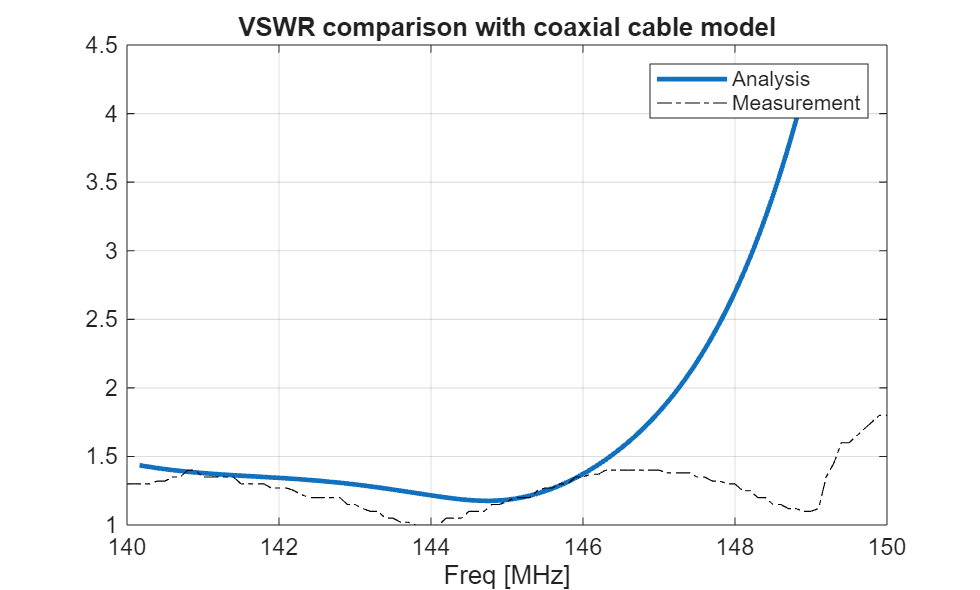The analysis VSWR curve for the coax and yagi antenna combination compares favorably with the measured data.

The results of the optimized design compare favorably with the fabricated antenna. This antenna will be used as part of a repeater station operating at 145 MHz.

figure
yagidesign.Tilt = 90;
yagidesign.TiltAxis = [0 1 0];
show(yagidesign)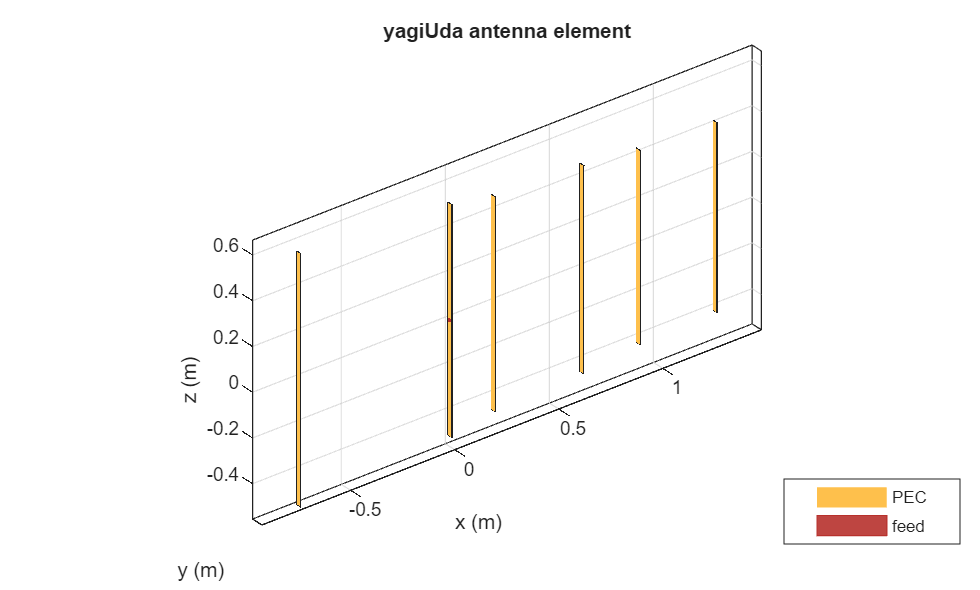%%